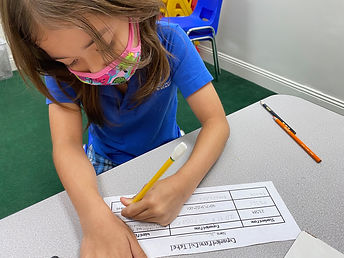## Ms. Dawn

### Target 1​

###### Lesson Type:

New

Number Operation

:

Integer Composition

Understand that the digits in a multi-digit number represent the amounts in each place-value.

###### 1:

Represent a number in written form (i.e., 1,234 is one thousand two hundred and thirty-four).

###### 2:

Understand the decimal point is written as “and”.

###### 3:

Identify place-value for a decimal number.

###### 4:

Represent a multi-digit number in expanded format.

4th

###### Vocabulary:

Word Form, Expanded Form, Standard Form, "And," Decimal point, Decimal number, Multi-digit number, Place-value, Worth

Activities:

1. Students verbally explained what each place value represented.

2. Students were given a chart that consisted of Standard Form, Word Form, and Expanded Form. They found the unknowns in the chart (ex. Given Standard Form- Translate it into Word Form and Expanded Form).

3. Students played a game by rolling a die and creating a varitey of decimal numbers. After rolling their numbers, They inputed into the chart and needed to solve and fill in the Expanded Form and Word Form. Students also verbally answered questions I asked as they worked (ex. read for me the number, how much is this number worth?),### Home Exploration

###### Guiding Questions:## Absent Students:

### Target 2

:

###### 1:

Use currency symbols (\$ and ₵) appropriately.

###### 2:

Add and subtract currency with decimal points.

###### Vocabulary:

Currency, Decimal point, "And"

Activities:

1. Students were given money and needed to figure out the total amount they had by adding it up.

2. Students were given pictures of a variety of amounts of money. They needed to figure out how much they had by adding it up.

3. Students had to verbally tell me how much money the pictures showed. They needed to use the vocabulary correctly (ex. \$5.67- needed to say five dollars "and" sixty-seven cents).### Home Exploration

###### Guiding Questions:### Target 3

:

###### 1:

Understand how to use place-value (the value of each digit) to compare numbers.

###### 2:

Order a set of integers from least to greatest (and greatest to least).

###### 3:

Compare decimals (up to the hundredths place).

###### 4:

When comparing decimals, understand to look at the greatest place-value first.

###### 5:

Explain how a number could have a lesser value, even if it has more digits than another (i.e., 4.33<5.2).

###### Vocabulary:

Place-value, Least, Greatest, Integers, Comparing, Lesser value, Value

Activities:

1. Students were given a variety of decimal numbers on a whiteboard. They needed to number it from least to greatest.

2. Students needed to show their understanding of comparing decimal numbers by completing a maze from start (least) to finish (greatest). They needed to get their lost alien to his planet.

3. Students verbally explained why a given decimal number was smaller or bigger than the other.### Home Exploration